# Difference between revisions of "Liu hard disk equation of state"

The Liu equation of state for hard disks (2-dimensional hard spheres) is given by Eq. 1 of .

For the stable fluid: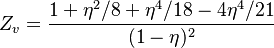$Z_v = \frac{1 + \eta^2/8 + \eta^4/18 - 4 \eta^4/21}{(1-\eta)^2}$

where the packing fraction is given by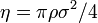$\eta = \pi \rho \sigma^2 /4$ where$\sigma$ is the diameter of the disks.

The EoS for the stable fluid, liquid-hexatic transition region and hexatic: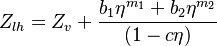$Z_{lh} = Z_v + \frac{b_1 \eta^{m_1} + b_2 \eta^{m_2}}{(1-c \eta)}$

The global EoS for all phases: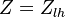$Z=Z_{lh}$,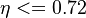$\eta <= 0.72$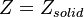$Z=Z_{solid}$,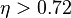$\eta > 0.72$

where: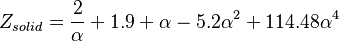$Z_{solid} = \frac{2}{\alpha} + 1.9 + \alpha - 5.2 \alpha^2 + 114.48 \alpha^4$

and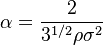$\alpha = \frac{2}{3^{1/2} \rho \sigma^2}$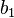$b_1$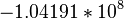$- 1.04191 * 10^8$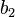$b_2$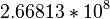$2.66813 * 10^8$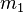$m_1$ 53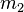$m_2$ 56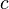$c$ 0.75